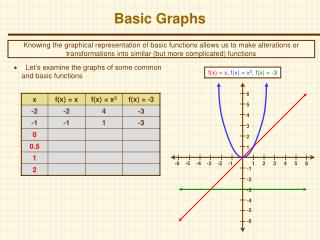# Basic Graphs - PowerPoint PPT PresentationDownload PresentationBasic Graphs

Basic GraphsDownload Presentation## Basic Graphs

- - - - - - - - - - - - - - - - - - - - - - - - - - - E N D - - - - - - - - - - - - - - - - - - - - - - - - - - -
##### Presentation Transcript

1. Basic Graphs Knowing the graphical representation of basic functions allows us to make alterations or transformations into similar (but more complicated) functions • Let’s examine the graphs of some common and basic functions f(x) = x, f(x) = x2, f(x) = -3 6 5 4 3 2 1 -6 -5 -4 -3 -2 -1 1 2 3 4 5 6 -1 -2 -3 -4 -5 -6

2. Basic Graphs Knowing the graphical representation of basic functions allows us to make alterations or transformations into similar (but more complicated) functions f(x) = |x|, f(x) = sqrt(x), f(x) = x3 6 5 4 3 2 1 -6 -5 -4 -3 -2 -1 1 2 3 4 5 6 -1 -2 -3 -4 -5 -6

3. Transformations • Now let’s review some basic transformations and their effects • Basically, the question becomes what is being affected by the addition / multiplication of a constant • The x variable (just the independent variable) => horizontal effect • The y variable (or f(x) as a whole) => vertical effect

4. Vertical Shifts • Vertical shifts (the “y” or entire function is changed by some constant) • The graph of y = f(x) + c is the graph of y = f(x) shifted up vertically by c units • The graph of y = f(x) - c is the graph of y = f(x) shifted down vertically by c units 6 5 4 3 2 1 -6 -5 -4 -3 -2 -1 1 2 3 4 5 6 -1 -2 -3 -4 -5 -6

5. Horizontal Shifts • Horizontal shifts (the “x” or independent variable is changed by some constant) • The graph of y = f(x + c) is the graph of y = f(x) shifted to the left (opposite the sign) by c units • The graph of y = f(x - c) is the graph of y = f(x) shifted to the right (opposite the sign) by c units 6 5 4 3 2 1 -6 -5 -4 -3 -2 -1 1 2 3 4 5 6 -1 -2 -3 -4 -5 -6

6. Example Graphs Horizontal Shifts Vertical Shifts f(x) = x - 2, g(x) = x2 + 1 f(x) = (x – 3)2,g(x) = sqrt(x + 1), h(x) = (x – 3)2 - 2 6 6 5 5 4 4 3 3 2 2 1 1 -6 -5 -4 -3 -2 -1 1 2 3 4 5 6 -6 -5 -4 -3 -2 -1 1 2 3 4 5 6 -1 -1 -2 -2 -3 -3 -4 -4 -5 -5 -6 -6

7. Reflections (graph is the same but reflected about the x / y axis) The graph of y = -f(x) is the graph of y = f(x) reflected about the x-axis (y values are changing) The graph of y = f(-x) is the graph of y = f(x) reflected about the y-axis (we’re changing the independent variable x) Reflections 6 5 4 3 2 1 -6 -5 -4 -3 -2 -1 1 2 3 4 5 6 -1 -2 -3 -4 -5 -6

8. Stretching / shrinking (graph is the stretched or shrunk vertically / horizontally) The graph of y = cf(x) is the graph of y = f(x) vertically stretched (multiplies y-coordinates by c) Think of grabbing the top and bottom of the graph and stretching it The graph of y = f(cx) is the graph of y = f(x) horizontally stretched (mult. x-coordinates by c) Stretching / Shrinking 6 5 4 3 2 1 -6 -5 -4 -3 -2 -1 1 2 3 4 5 6 -1 -2 -3 -4 -5 -6

9. Example Graphs Reflections Stretching / Shrinking f(x) = -sqrt(x), g(x) = -x3, h(x) = sqrt(-x) f(x) = 2sqrt(x), g(x) = sqrt(2x), h(x) = ½x2 6 6 5 5 4 4 3 3 2 2 1 1 -6 -5 -4 -3 -2 -1 1 2 3 4 5 6 -6 -5 -4 -3 -2 -1 1 2 3 4 5 6 -1 -1 -2 -2 -3 -3 -4 -4 -5 -5 -6 -6

10. Multiple Transformations Book problems: 53,55,59,63,67,69,77,81,83,87,95,100,103,107,109,115 • These basic transformations can be applied to functions (graphs) individually or in combination with one another • Look at a couple of HW exercises as an example 6 6 5 5 4 4 3 3 2 2 1 1 -6 -5 -4 -3 -2 -1 1 2 3 4 5 6 -6 -5 -4 -3 -2 -1 1 2 3 4 5 6 -1 -1 -2 -2 -3 -3 -4 -4 -5 -5 -6 -6